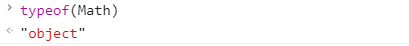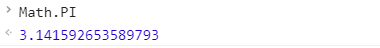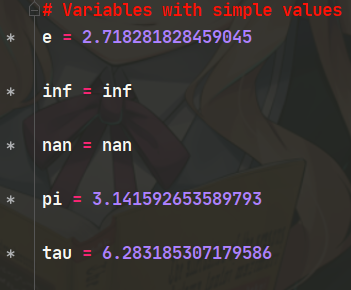• python函数必须import math 返回x的反余弦、返回x的反双曲余弦、返回x的反正玄、返回x的反正切、返回y\x的反正切python教程
• 1、math模块-数论和表示函数（Number-Theoretical and Representation Functions) 函数 使用说明 ceil(x) 取大于等于x的最小的整数值，如果x是一个整数，则返回x copysign(x,y) 把y的正负号加到x前面...
1、math模块-数论和表示函数（Number-Theoretical and Representation Functions)
函数使用说明ceil(x)取大于等于x的最小的整数值，如果x是一个整数，则返回xcopysign(x,y)把y的正负号加到x前面，可以使用0fabs(x)返回x的绝对值（float类型）factorial(x)取x阶乘的值floor(x)取小于等于x的最大整数值，如果x是一个整数，返回自身fmod(x,y)得到x/y的余数，返回float类型frexp(x)返回一个元组(m,e)，其计算方式为：x分别除以0.5和1，得到一个值的范围fsum(iterable)对迭代器里的每个元素进行求和操作gcd(x,y)返回x和y的最大公约数isclose(a,b,*,rel_tol=1e-09,abs_tol=0.0)如果值a和彼此接近，则返回True，否则返回False；rel_tol是相对容差-它是a和b之间允许的最大差值。abs_tol是最小绝对容差---对于接近0的比较有用isfinite(x)如果x既不是无穷也不是NAN,则返回True；否则返回Falseisinf(x) 如果x是正无穷大或负无穷大，则返回True,否则返回False isnan(x)如果x不是数字，返回Trueldexp(x)返回x*(2**i)modf(x)返回由x的小数部分和整数部分组成的元组trunc(x)返回x的整数部分
2、math模块-幂数和对数函数（Power and Logarithmic Functions)
函数使用说明exp(x）返回e**xexpm1(x)返回e**(x-1)log(x[,base])返回x的自然对数，默认以e为基数；base参数给定时，将x的对数返回给定的baselog1p(x)返回1+x的自然对数（基数e)。计算结果的方式对于接近零的x是准确的log2(x)返回x基数为2的对数。通常比log(x,2)更准确log10(x)返回x基数为10的对数。通常比log(x,10)更准确pow(x,y)返回x的y次方，即x**ysqrt(x)返回x的平方根
3、math模块-数学常量（Constants）
常量使用说明pi数学常量Π=3.1415926...，可用精度e数学常量e=2.718281...，可用精度tau数学常量=6.283185···,可用精度。Tau是一个等于2的圆常量，即圆的周长与其半径之比inf浮点正无限。（对于负无穷大，请使用math.inf）等同于float（‘inf')的输出nan浮点“不是数字”（NaN)值。等同于float('nan')的输出。


展开全文• ## C# Math函数汇总

千次阅读 2021-06-18 08:58:18
Math类中的常用函数 功能 函数 示例 数学表示 描述 乘方 Math.Pow() Math.Pow(2,2) 返回指定数字的指定次幂 开方 Math.Sqrt() Math.Sqrt(16) 返回指定数字的平方根 e的次方 Math.Exp() Math.Exp(8) 返回 e 的指定次...
Math类中的常用函数
功能函数示例数学表示描述乘方Math.Pow()Math.Pow(2,2)返回指定数字的指定次幂开方Math.Sqrt()Math.Sqrt(16)返回指定数字的平方根e的次方Math.Exp()Math.Exp(8)返回 e 的指定次幂绝对值Math.Abs()Math.Abs(-3)返回有符号整数的绝对值对数Math.Log()Math.Log(8,2)返回指定数字在使用指定底时的对数常用对数Math.Log10()Math.Log10(100)返回指定数字以 10 为底的对数正弦函数Math.Sin()Math.Sin(Math.PI/3)返回指定角度的正弦值余弦函数Math.Cos()Math.Cos(Math.PI/3)返回指定角度的余弦值正切函数Math.Tan()Math.Tan(Math.PI/3)返回指定角度的正切值

Math类中的常用数
数学含义近似值代码表示代码描述3.1415926535897931Math.PI表示圆的周长与其直径的比值，它通过常数 n 指定e2.7182818284590451Math.E表示自然对数的底，它由常数 e 指定


展开全文c#
• 数学函数Math是对象数据类型 ,里边提供了许多数学函数方法 常用方法： 1、Math.abs 取绝对值 (正数) Math.abs(12) -> 12 Math.abs(-12) -> 12 2、Math.ceil 向上取整 Math.ceil(12) -> 12 Math...
数学函数： Math是对象数据类型 ,里边提供了许多数学函数方法常用方法：
1、Math.abs  取绝对值 (正数)
Math.abs(12) -> 12
Math.abs(-12) -> 12
2、Math.ceil 向上取整
Math.ceil(12) -> 12
Math.ceil(12.1) -> 13
Math.ceil(12.9) -> 13
3、Math.floor 向下取整
Math.floor(12) -> 12
Math.floor(12.1) -> 12
Math.floor(12.9) -> 12
4、Math.round 四舍五入
Math.round(12.3) -> 12
Math.round(12.7) -> 13
5、Math.random  获取 【0,1) 之间的随机小数，左闭右开 -  包括0，不包括1
（1）获取1到10 的正数：
// Math.random()*10 获取0到10 之前的小数，不包含10
// Math.round() 把上边小数四舍五入 - 即可获得0到10 之间的整数
Math.round(Math.random()*10)
（2）获取3到15 之间的整数：
//  找规律： 想获取 3 到15之间的数，只需要 Math.random()*12得到 1到12，然后
//  加 3 即可
//  规律： Math.random(最大值 - 最小值)得到0到 （最大值-最小值）之间的数，然后加最小值
//  即可得到 最小值到最大值 之间的整数
Math.round(Math.random()*12 +3)
( 3 ) 获取 min最小值，到max最大值之间的整数
Math.round(Math.random()*(max-min) + min)
6、Math.PI  圆周率7、Math.pow( ) 获取一个值的多少次幂
Math.sqrt( ) 开平方
Math.pow(10, 2)  -> 10的2次方 -> 100
Math.sqrt(100) -> 100开平方 -> 10
展开全文• math 完整函数 详细介绍 java math包含的各种函数
• 文章目录Python 标准库之 Math 数学函数导入模块Math 常量math.pimath.emath.taumath.infmath.nanmath.ceil(x)math.floor(x)math.comb(n, k)math.copysign(x, y)math.fabs(x)math.factorial(x)math.fmod(x, y)math....Python 标准库之 Math 数学函数

文章目录
Python 标准库之 Math 数学函数导入模块🦹‍♂️一、Math 常量📐1) math.pi2) math.e3) math.tau4) math.inf5) math.nan
二、Math 表示函数🎏1) math.ceil(x) 常用2) math.floor(x) 常用3) math.comb(n, k)4) math.copysign(x, y)5) math.fabs(x) 绝对值6) math.factorial(x) 阶乘7) math.fmod(x, y) 取模8) math.frexp(x)9) math.fsum(iterable)10) math.gcd(a, b) 最大公约数11) math.isclose(a, b, rel_tol=1e-09, abs_tol=0.0)12) math.isinf(x)13) math.isnan(x)14) math.isfinite(x)15) math.isqrt(n) 平方根16) math.ldexp(x, i)17) math.modf(x)18) math.perm(n, k=None)19) math.prod(iterable, start=1)20) math.remainder(x, y)21) math.trunc(x)
三、Math 幂与对数math.exp(x)math.expm1(x)math.log(x[, base])math.log1p(x)math.log2(x)math.log10(x)math.pow(x, y)math.sqrt(x)
四、Math 三角函数math.acos(x)math.asin(x)math.atan(x)math.atan2(y, x)math.cos(x)math.dist(p, q)
相关博客😏

导入模块🦹‍♂️
import math

math 为标准库下的模块，直接导入即可

一、Math 常量📐
常量部分源码👇1) math.pi
返回 圆周率 π = 3.141592…，精确到 小数点后 15 位
pi = math.pi

2) math.e
返回数学常数 e = 2.718281…，精确到 小数点后 15 位
e = math.e

3) math.tau
返回数学常数 τ = 6.283185…，精确到 小数点后 15 位。 Tau 是一个圆周常数，等于 2π，圆的周长与半径之比。
tau = math.tau

4) math.inf
表示 浮点正无穷大 ，要表示负无穷大，得使用 -math.inf ，相当于 float(‘inf’)、float("-inf") 的输出
正无穷大：inf负无穷大：-inf
Positive_infinity = math.inf
Negative_infinity = -math.inf

5) math.nan
表示 NaN : Not a Number（非数字） 代表不是一个数字，浮点数类型，相当于 float(‘nan’) 的输出，通常只用于表示缺少某些数据

二、Math 表示函数🎏
1) math.ceil(x) 常用
返回 x 的上限值

x 为浮点数时则 向上取整，为整数时直接返回，不做任何更改
num1 = 5.668
num2 = 5
print(math.ceil(num1))   # 结果为 6
print(math.ceil(num2))   # 结果为 5

2) math.floor(x) 常用
返回 x 的向下取整

x 为浮点数时则 向下取整，为整数时直接返回，不做任何更改
num1 = 5.668
num2 = 4
print(math.floor(num1))   # 结果为 5
print(math.floor(num2))   # 结果为 4

3) math.comb(n, k)
返回 不重复且无顺序地 从 n 项中选择 k 项的方式总数 当 k <= n 时取值为『n! / (k! * (n - k)!)』，当 k > n 时取值为零。也称为二项式系数，因为它等价于表达式 (1 + x) ** n 的多项式展开中第 k 项的系数。
图解👇print(math.comb(4, 2))     # 结果为 6


如果任一参数不为整数则会引发 TypeError，如果任一参数为负数则会引发 ValueError。

4) math.copysign(x, y)
copysign 从字面上理解，中文释义的为 “复制符号”

返回一个 基于** x 的绝对值** 和 y 的符号 的 浮点数，就是先获取 x 绝对值，再看 y 是什么符号，将 y 的符号给 x 的绝对值，x 可以是 整数 或则 浮点数，但最后返回的值为浮点数类型。 在支持 带符号 零(-0) 的平台上，copysign(1.0, -0.0) 返回 -1.0
print(math.copysign(6.6, -2))    # 结果为 -6.6
print(math.copysign(6.6, 2))     # 结果为 6.6
print(math.copysign(6.6, -2.6))  # 结果为 -6.6
print(math.copysign(6.6, 2.6))   # 结果为 6.6
print(math.copysign(5, -2.6))    # 结果为 -5.0
print(math.copysign(5, 2))       # 结果为 5.0

5) math.fabs(x) 绝对值
返回 x 的绝对值，x 可以是 整数 或者 浮点数，返回的值为浮点数类型
print(math.fabs(-6))    # 结果为 6.0

6) math.factorial(x) 阶乘
以 一个整数 返回 x 的阶乘，如果 x 不是整数 或为 负数 时则将引发 ValueError
print(math.factorial(4))         # 4的阶乘为 24
print(math.factorial(5))         # 5的阶乘为 120

7) math.fmod(x, y) 取模
取模，返回除法的余数，math.fmod(x, y) 与运算符 x % y 公式相同『 x - (x / y)*x 』，但区别在 (x / y) 上的取值方式不同，x % y 将 ( x / y) 的值向下取整，而 math.fmod(x, y) 将 (x / y) 的值向0取整
图解👇print(3 % 2)             # 结果为 1
print(math.fmod(3, 2))   # 结果为 1.0
print(-3 % 2)            # 结果为 1
print(math.fmod(-3, 2))  # 结果为 -1.0


math.fmod 返回的是浮点数类型

8) math.frexp(x)
以 (m, e) 对的形式返回 x 的 尾数 和 指数，其算式为 『 x == m * 2**e 』 ，本质是 以 2为基数 的科学计数法， m 是一个浮点数， e 是一个整数 如果 x 为零，则返回 (0.0, 0) ，否则返回 0.5 <= abs(m) < 1
print(math.frexp(300))      # 结果为 (0.5859375, 9)
print(math.frexp(-300))     # 结果为 (-0.5859375, 9)
print(0.5859375 * 2 ** 9)   # 0.5859375为尾数，2为基数，9为指数

9) math.fsum(iterable)
iterable 为一个可迭代对象，如：列表、元组、集合，将数组内的值进行求和计算，此方法功能与 内置函数sum() 相同，但比 sum() 能获取计算精确浮点值，返回的求和值为 浮点数类型。
print(sum([0.1] * 10))        # 结果为 0.9999999999999999
print(math.fsum([0.1] * 10))  # 结果为 1.0
print(math.fsum([6, 60]))     # 结果为 66.0

10) math.gcd(a, b) 最大公约数
返回 a 和 b 的 最大公约数，a 和 b 只能是整数，gcd(0, 0) 返回 0。
print(math.gcd(18, 66))     # 结果为 6
print(math.gcd(6, 7))       # 结果为 1
print(math.gcd(0, 6))       # 结果为 6
print(math.gcd(0, 0))       # 结果为 0

11) math.isclose(a, b, rel_tol=1e-09, abs_tol=0.0)
若 a 和 b 的值 比较接近则返回 True，否则返回 False，根据给定的 绝对 和 相对 容差确定两个值是接近。
rel_tol 是相对容差 ，它是 a 和 b 之间允许的最大差值，相对于 a 或 b 的较大绝对值。例如，要设置5％的容差，请传递 rel_tol=0.05， rel_tol 必须大于零，默认容差为 1e-09（科学计数法，即为 1 乘 10的 -9 次方）。abs_tol 是最小绝对容差，对于接近零的比较很有用。 abs_tol 必须至少为零。
判断是否接近的公式为： abs(a-b) <= max(rel_tol * max(abs(a), abs(b)), abs_tol)

abs() 函数返回数字的绝对值。 max() 方法返回给定参数的最大值，参数可以为序列。

图解👇print(math.isclose(50, 52.6, rel_tol=0.05))    # 结果为 True
print(math.isclose(50, 52.7, rel_tol=0.05))    # 结果为 False

具体来说， NaN 不被认为接近任何其他值，包括 NaN，inf 和 -inf 只被认为接近自己
print(math.isclose(math.nan, math.nan))     # 结果为 False
print(math.isclose(math.inf, math.inf))     # 结果为 True
print(math.isclose(math.inf, -math.inf))    # 结果为 False

12) math.isinf(x)
判断 x 是否为 正或负无穷大，是则返回 True ，否则返回 False
正无穷大：inf负无穷大：-inf
print(math.isinf(float("inf")))  # 结果为 True
print(math.isinf(math.inf))      # 结果为 True
print(math.isinf(-math.inf))     # 结果为 True
print(math.isinf(66))            # 结果为 False

13) math.isnan(x)
判断 x 是否为 NaN，是则返回 True ，否则返回 False NaN : Not a Number（非数字） 代表不是一个数字，浮点数类型
print(math.isnan(float("nan")))		# 结果为 True
print(math.isnan(math.nan))			# 结果为 True
print(math.isnan(6))				# 结果为 False

14) math.isfinite(x)
判断 x 是否为有限的，如果 x 既 不是无穷大 也 不是NaN，则返回 True ，否则返回 False
print(math.isfinite(0.0))           # 结果为 True
print(math.isfinite(10 ** 100))     # 结果为 True
print(math.isfinite(float("inf")))  # 结果为 False
print(math.isfinite(math.inf))      # 结果为 False
print(math.isfinite(math.nan))      # 结果为 False

15) math.isqrt(n) 平方根
返回 非负整数 n 的 整数平方根，对 n 的实际平方根向下取整
print(math.isqrt(9))            # 结果为 3
print(math.isqrt(6))            # 结果为 2
print(math.isqrt(3))            # 结果为 1
print(1 + math.isqrt(3 - 1))    # 先上取整写法


如果 n 为负数，会抛出ValueError异常

16) math.ldexp(x, i)
返回 x * (2 ** i) 的 浮点数值 ， 这基本上是函数 frexp() 的反函数
x = 66
m, i = math.frexp(x)            # m为尾数，i为指数
print(math.ldexp(m, i) == x)	# 结果为 True

17) math.modf(x)
返回 x 的 小数、整数 部分的数组，相当于对小数点前后进行了拆分，返回的值都 带有 x的符号，且都是浮点数类型
x = math.modf(5.6)
print(x)                        # 小数部分存在精确不足的问题
print(round(x, 1), x[-1])    # 可以使用round() 解决

(0.5999999999999996, 5.0)
0.6 5.0

18) math.perm(n, k=None)
返回不重复且有顺序地从 n 项中选择 k 项的方式总数，此方法类似于 comb()，但内部算式不同 当 k <= n 时取值为 『n! / (n - k)! 』；当 k > n 时取值为零。
图解👇print(math.comb(4, 2))  # 结果为 6
print(math.perm(4, 2))  # 结果为 12

如果 k 未指定或为 None，则 k 默认值为 n 并且函数将返回 n!（n 的阶乘）
print(math.perm(4, 4))
print(math.perm(4))

24
24


如果任一参数不为整数则会引发 TypeError。 如果任一参数为负数则会引发 ValueError。

19) math.prod(iterable, start=1)
计算输入的 iterable 中所有元素的 积
iterable：为一个可迭代对象，如：列表、元组、集合start：相当于 求得 iterable 中所有元素的积 再乘于 start，默认值为 1

类似于 内置函数 sum(iterable, start=0)，将 iterable 中所有元素求和，再加上 start

print(math.prod([1, 2, 3, 4, 5, 6]))               # 结果为 720
print(math.prod([1, 2, 3, 4, 5, 6], start=0.5))    # 结果为 360.0

当可迭代对象为空时，返回起始值
print(math.prod([]))    # 结果为 1

20) math.remainder(x, y)
返回 x 相对于 y 的余数，对于有限 x 和有限非零 y ，计算公式为： x - n * y ，其中 n 为 x / y 的精确值最接近的整数 (应该是四舍五入)
print(math.remainder(3, 2))
print(math.remainder(6, 4))
print(math.remainder(6, 5))

-1.0
-2.0
1.0

remainder(x, math.inf) 对于任何有限 x 都是直接返回 x
print(math.remainder(6, math.inf))     # 结果为 6.0


remainder(x, 0) 和 remainder(math.inf, x) 引发 ValueError

21) math.trunc(x)
截取 x 的整数部分并返回
print(math.trunc(6.66666666666666666))  # 结果为6
print(math.trunc(623e-2))               # 科学计数法，实则6.23
print(math.trunc(6.0))                  # 结果为6
print(math.trunc(6))                    # 结果为6


三、Math 幂与对数
math.exp(x)
返回 e 次 x 幂，其中 e = 2.718281… 是自然对数的基数。这通常比 math.e ** x 或 pow(math.e, x) 更精确。
math.expm1(x)
返回 e 的 x 次幂，减1。这里 e 是自然对数的基数。对于小浮点数 x ， exp(x) - 1 中的减法可能导致 significant loss of precision； expm1() 函数提供了一种将此数量计算为全精度的方法:
math.log(x[, base])
使用一个参数，返回 x 的自然对数（底为 e ）。
使用两个参数，返回给定的 base 的对数 x ，计算为 log(x)/log(base) 。
math.log1p(x)
返回 1+x (base e) 的自然对数。以对于接近零的 x 精确的方式计算结果。
math.log2(x)
返回 x 以2为底的对数。这通常比 log(x, 2) 更准确。
math.log10(x)
返回 x 底为10的对数。这通常比 log(x, 10) 更准确。
math.pow(x, y)
将返回 x 的 y 次幂。特殊情况尽可能遵循C99标准的附录’F’。特别是， pow(1.0, x) 和 pow(x, 0.0) 总是返回 1.0 ，即使 x 是零或NaN。 如果 x 和 y 都是有限的， x 是负数， y 不是整数那么 pow(x, y) 是未定义的，并且引发 ValueError 。
与内置的 ** 运算符不同， math.pow() 将其参数转换为 float 类型。使用 ** 或内置的 pow() 函数来计算精确的整数幂。
math.sqrt(x)
返回 x 的平方根。

四、Math 三角函数
math.acos(x)
以弧度为单位返回 x 的反余弦值。
math.asin(x)
以弧度为单位返回 x 的反正弦值。
math.atan(x)
以弧度为单位返回 x 的反正切值。
math.atan2(y, x)
以弧度为单位返回 atan(y / x) 。结果是在 -pi 和 pi 之间。从原点到点 (x, y) 的平面矢量使该角度与正X轴成正比。 atan2() 的点的两个输入的符号都是已知的，因此它可以计算角度的正确象限。 例如， atan(1) 和 atan2(1, 1) 都是 pi/4 ，但 atan2(-1, -1) 是 -3*pi/4 。
math.cos(x)
返回 x 弧度的余弦值。
math.dist(p, q)
返回 p 与 q 两点之间的欧几里得距离，以一个坐标序列（或可迭代对象）的形式给出。 两个点必须具有相同的维度。

相关博客😏
Python 计算平方根
展开全文python 数学
• ## C#中Math类常用函数

千次阅读 2017-09-07 19:40:25c#
• Math包含用于执行基本数字运算的方法 算术运算 Math.abs(a)：取a的绝对值 Math.sqrt(a)：取a的平方根 Math.cbrt(a)：取a的立方根 Math.max(a,b)：取a、b之间的最大值 Math.min(a,b)：取a、b之间的最小值 Math....java
• math标准函数库概述 math库一共提供了4个...44个函数分为4类，包括：16个数值表示函数、8个幂对数函数、16个三角对数函数和四个高等特殊函数 是Python提供内置数学类函数库 在使用math库前，用import导入该...python
• ## js中Math常用的函数

千次阅读 2019-08-27 19:50:04
其中，Math对象封装了许多常用的数学函数Math.random 该方法用于生成一个随机数0~1 包含0 不包含1 // 获取一个从0到9的随机整数数字 var r = Math.random(); var num = parseInt(Math.random() * 10);对于...
• 函数 描述 格式 返回值 ceil() 向上取整操作 math.ceil(数值) 整型 floor() 向下取整操作 (对比内置round) math.floor(数值)) 整型 pow() 计算一个数值的N次方 math.pow(底数,指数) 浮点型 sqrt() 开平方...python 数学
• 三角函数包括反三角函数的实现，基本思想是利用泰勒级数。鉴于反三角函数ACOS在0.9-1的时候利用直接利用泰勒级数时收敛很慢的缺点，比较ulicx作了优化，使得精度和时间都达到了自己预期。适用于在嵌入式环境下不好...
• ## math.h 包含函数介绍

万次阅读 多人点赞 2016-04-26 17:28:35
数学函数库，一些数学计算的公式的具体实现是放在math.h里，具体有： 1、 三角函数 double sin(double);正弦 double cos(double);余弦 double tan(double);正切 2 、反三角函数 double asin ...
• Math.random()函数返回0和1之间的伪随机数，可能为0，但总是小于1，[0,1) 生成n-m，包含n但不包含m的整数： 第一步算出 m-n的值，假设等于w 第二步Math.random()*w 第三步Math.random()*w+n 第四步parseInt(Math....
• 这个单元包含高性能的算术、三角、对数、统计和金融方面的计算及FPU程序函数用于补充Delphi语言System.pas 单元中的数学常规程序函数 注意 1) 对于有些函数，这个单元中涉及的概念或常数由Earl F. Glynn ...
• Math 是数学函数，但又属于对象数据类型 typeof Math => ‘object’ console.dir(Math) 查看Math的所有函数方法。 1，Math.abs() 获取绝对值 Math.abs(-12) = 12 2，Math.ceil() and Math.floor() 向上取整和向...javascript html vue.js css 前端
• Math的官方函数 1.Math.random() 作用：随机产生0-1之间的数,不包括1 var t = parseInt(Math.random()*10); 随机取出0~10之间的一个整数，不包括10 2.Math.round(n) 作用：四舍五入取整 Math.round(4.2);结果是4 3....javascript
• C语言提供了很多实用的数学函数，如果要使用，需要在程序开头加上math.h头文件。下面是几个比较常用的数学函数，需要掌握。 1.fabs(double x)/abs(int x)对变量x取绝对值。 2.floor(double x)对变量x向下取整，如...
• 注：以下的有关函数及头文件参考了网上资料 #include <stdlib.h> int abs( int num ); double fabs( double arg ); long labs( long num ); 函数返回num的绝对值 #include <stdlib.h> div_t div( int...C语言 库函数
• JavaScript Math内置函数 /* Math 对象 pow(x,y) 返回 x 的 y 次幂 sqrt(x) 返回 x 的平方根 */ console.log(Math); console.log(Math.PI); var n = Math.abs(-10); console.lo...JavaScript
• Math：数学函数。虽然叫函数，但是Math是对象数据类型的。...输入console.dir(Math)就知道Math包含着很多方法。 如下图所示，我只是简单的列举了一些Math中自带的方法，有一些是不常用的。 下面我就介绍10javascript
• math库常用函数及举例： 注意：使用math库前，用import导入该库>>> import math 取大于等于x的最小的整数值，如果x是一个整数，则返回x>>> math.ceil(4.12)5 把y的正负号加到x前面，可以...
• 文章目录一、math.h二、常用函数举例求绝对值求两者中最大/小值求指数/对数等三、函数名中的规律 一、math.h 这篇博文记录一下C语言中常用的一个数学函数库，里面涵盖了常用的数学运算，包括对...math包括很多函数c语言
• ## java 的Math函数库

千次阅读 2019-08-07 14:35:12
java.math是一个包，提供用于...java.lang.Math类中包含E和PI两个静态常量，以及进行科学计算的类(static)方法，可以直接通过类名调用。 public static final Double E = 2.7182818284590452354 public static f...
• Math函数方法有很多,用 console.dir(Math) 可以在浏览器控制台中查看所有的Math方法. 这里主要整理一下常用的Math函数方法 1、 Math.ceil() and Math.floor() 向上取整和向下取整。 (ceil有天花板的意思,向上;floor...js javascript
• 1. math.fabs(x): 返回x的绝对值。同numpy。 >>> import numpy >>> import math >>> numpy.fabs(-5) 5.0 >>> math.fabs(-5) 5.0 2.x.astype(type): 返回type类型的x, type ...python numpy
• 求取绝对值的函数 intabs(int i);//返回整型数i的绝对值； 例如int abs(-3.5) == 3.5; //double cabs(struct complex znum);//返回复数znum绝对值； double fabs(double x);//返回双精度数x的绝对值；例如double ...
•  math 模块实现了正常情况下内置平台 C 库中才有的很多 IEEE 函数，可以使用浮点值完成复杂的数学运算，包括对数和三角函数运算。 特殊常量  很多数学运算依赖于一些特殊的常量。math 包含有 π（pi...